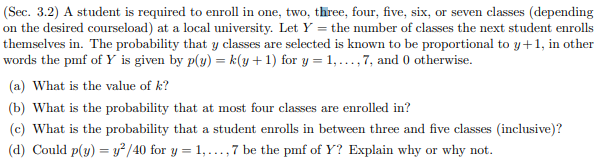# (Sec. 3.2) A student is required to enroll in one, two, three, four, five, six on the desired courseload) at a local university. Let Y the number of classes the next student enrolls themselves in. The probability that y classes are selected is known to be proportional to y+1, in other words the pmf of Y is given by p(y) = k(y+1) for y 1,...,7, and 0 otherwise (a) What is the value of k? or seven classes (depending (b) What is the probability that at most four classes are enrolled in? (c) What is the probability that a student enrolls in between three and five classes (inclusive)? y? /40 for y 1,.,7 be the pmf of Y? Explain why why not (d) Could p(y) or

Questionhelp_outlineImage Transcriptionclose(Sec. 3.2) A student is required to enroll in one, two, three, four, five, six on the desired courseload) at a local university. Let Y the number of classes the next student enrolls themselves in. The probability that y classes are selected is known to be proportional to y+1, in other words the pmf of Y is given by p(y) = k(y+1) for y 1,...,7, and 0 otherwise (a) What is the value of k? or seven classes (depending (b) What is the probability that at most four classes are enrolled in? (c) What is the probability that a student enrolls in between three and five classes (inclusive)? y? /40 for y 1,.,7 be the pmf of Y? Explain why why not (d) Could p(y) or fullscreen

### Want to see this answer and more?

Experts are waiting 24/7 to provide step-by-step solutions in as fast as 30 minutes!*